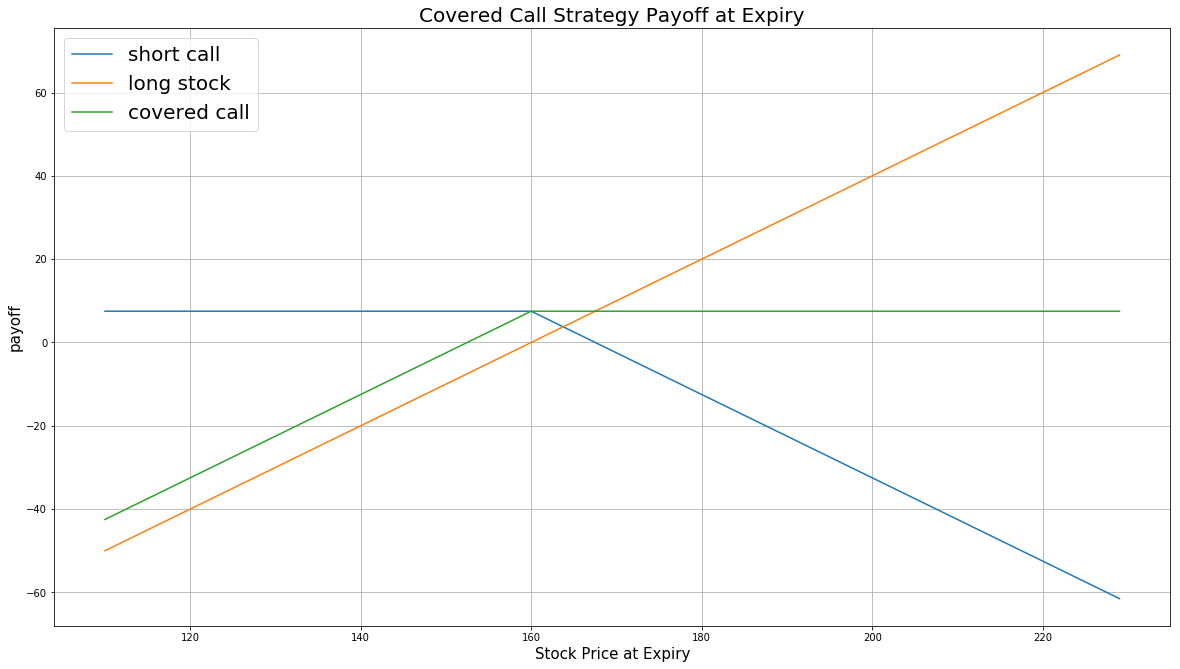# Option Strategies

## Covered Call

### Introduction

A Covered Call consists of buying a stock and selling call Options for the same amount of stock. The aim of the Covered Call is to profit from the Option premium by selling calls written on the stock you already owned. At any time for US Options or at expiration for European Options, if the stock moves below the strike price, you keep the premium and still maintain the underlying Equity position. If the underlying price moves above the strike, the Options contract will be exercised and you will have to sell the stock at the strike price, but you will still keep the premium. Another risk of a Covered Call comes from the long stock position, whose value could drop.

### Implementation

Follow these steps to implement the covered call strategy:

1. In the Initialize method, set the start date, set the end date, cash, subscribe to the underlying Equity, and create an Options universe.
2. private Symbol _equity;
private Symbol _symbol;

public override void Initialize()
{
SetStartDate(2016, 1, 1);
SetEndDate(2016, 3, 1);
SetCash(100000);

_symbol = option.Symbol;
option.SetFilter(universe => universe.Strikes(-3, 3)
.Expiration(TimeSpan.FromDays(0), TimeSpan.FromDays(30)));
}
def Initialize(self) -> None:
self.SetStartDate(2016, 1, 1)
self.SetEndDate(2016, 3, 1)
self.SetCash(100000)

self.symbol = option.Symbol
option.SetFilter(-3, +3, timedelta(0), timedelta(30))
3. In the OnData method, select the Option contract.
4. public override void OnData(Slice slice)
{
if (Portfolio.Invested) return;

// Get the OptionChain
var chain = slice.OptionChains.get(_symbol, null);
if (chain == null || chain.Count() == 0) return;

// Get the ATM strike price
var atmStrike = chain.OrderBy(x => Math.Abs(x.Strike - chain.Underlying.Price)).First().Strike;

// Select the ATM call Option contracts
var calls = chain.Where(x => x.Strike == atmStrike && x.Right == OptionRight.Call);
if (calls.Count() == 0) return;

// Select the contract with the furthest expiry
var contracts = calls.OrderBy(x => x.Expiry);
var contract = contracts.Last();
def OnData(self, slice: Slice) -> None:
if self.Portfolio.Invested: return

# Get the OptionChain
chain = slice.OptionChains.get(self.symbol, None)
if not chain: return

# Get the ATM strike price
atm_strike = sorted(chain, key = lambda x: abs(chain.Underlying.Price - x.Strike)).Strike

# Select the ATM call Option contracts
calls = [x for x in chain if x.Strike == atm_strike and x.Right == OptionRight.Call]
if len(calls) == 0: return

# Select the contract with the furthest expiry
contracts = sorted(calls, key=lambda x: x.Expiry, reverse=True)
contract = contracts
5. In the OnData method, sell the Option contract and buy the corresponding amount of shares in the underlying Equity.
6. Sell(contract.Symbol, 1); // Short the call Option
Buy(_equity, 100);        // Buy 100 shares (contract multiplier) of the underlying stock
self.Sell(contract.Symbol, 1) # Short the call Option
self.Buy("IBM", 100)          # Buy 100 shares (contract multiplier) of the underlying stock

### Strategy Payoff

The payoff of the strategy is

$$\begin{array}{rcll} C_T & = & (S_T - K)^{+}\\ P_T & = & (S_T - S_0 + C_0 - C_T)\times m - fee \end{array}$$ $$\begin{array}{rcll} \textrm{where} & C_T & = & \textrm{Call value at time T}\\ & S_T & = & \textrm{Underlying asset price at time T}\\ & K & = & \textrm{Call strike price}\\ & P_T & = & \textrm{Payout total at time T}\\ & S_0 & = & \textrm{Underlying asset price when the trade opened}\\ & C_0 & = & \textrm{Call price when the trade opened (credit received)}\\ & m & = & \textrm{Contract multiplier}\\ & T & = & \textrm{Time of expiration} \end{array}$$

The following chart shows the payoff at expiration:The maximum profit is $K - S_T + C_0$. It occurs when the underlying price is at or above the strike price of the call at expiration.

If the underlying price drop, the maximum loss is $S_0 - C_0$.

If the Option is American Option, there is risk of early assignment on the sold contract.

### Example

The following table shows the price details of the assets in the algorithm:

AssetPrice ($)Strike ($)
Call3.50185.00
Underlying Equity at start of the trade187.07-
Underlying Equity at expiration190.09-

Therefore, the payoff is

$$\begin{array}{rcll} C_T & = & (S_T - K)^{+}\\ & = & (190.09 - 185)^{+}\\ & = & 5.09\\ P_T & = & (S_T - S_0 + C_0 - C_T)\times m - fee\\ & = & (190.09 - 187.07 + 3.50 - 5.09)\times m - fee\\ & = & 141 \end{array}$$

So, the strategy gains \$141.

The following algorithm implements a covered call strategy:

You can also see our Videos. You can also get in touch with us via Discord.